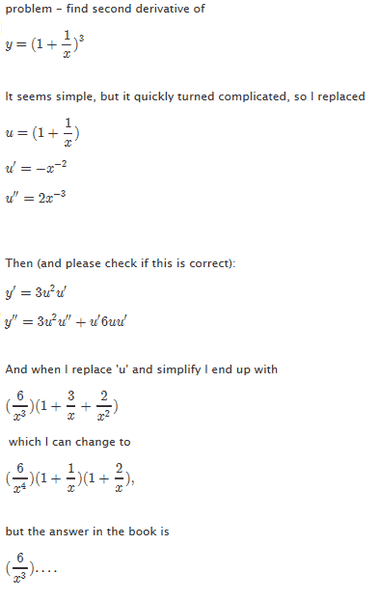# Problem - find second derivative

The problem and my attempt at a solution is shown in the attached image.

The problem is that I end up with one extra x in the denominator.
So the question is: Is my expression for y'' correct and I just made a mistake somewhere (I have checked it several times), or am I missing something in the expression for y' and/or y'' ?#### Attachments

MarneMath
If the answer in the book is something like 6/x^3 + 18/x^4 + 12/x^5, you got the same answer.

SammyS
Staff Emeritus
Homework Helper
Gold Member
The problem and my attempt at a solution is shown in the attached image.

The problem is that I end up with one extra x in the denominator.
So the question is: Is my expression for y'' correct and I just made a mistake somewhere (I have checked it several times), or am I missing something in the expression for y' and/or y'' ?

[ IMG]https://www.physicsforums.com/attachment.php?attachmentid=53971&stc=1&d=1355635814[/PLAIN]
How do you go from $\displaystyle\ \frac{6}{x^3}\left( 1+\frac{3}{x}+\frac{2}{x^2}\right)$/extract_itex] to $\displaystyle\ \frac{6}{x^4}\left( 1+\frac{1}{x}\right)\left( 1+\frac{2}{x}\right)\ ?$ $\displaystyle\ 1+\frac{3}{x}+\frac{2}{x^2}=\left( 1+\frac{1}{x}\right)\left( 1+\frac{2}{x}\right)\ .$ symbolipoint Homework Helper Education Advisor Gold Member Does this make sense? $$\[ \begin{array}{l} y' = 3(1 + {\textstyle{1 \over x}})^2 x^{ - 2} ( - 1) \\ = \frac{{ - 3(1 + {\textstyle{1 \over x}})^2 }}{{x^2 }} \\ \end{array}$$$

Next you would use quotient rule to find the next derivative (second derivative).

How do you go from $\displaystyle\ \frac{6}{x^3}\left( 1+\frac{3}{x}+\frac{2}{x^2}\right)\$ to $\displaystyle\ \frac{6}{x^4}\left( 1+\frac{1}{x}\right)\left( 1+\frac{2}{x}\right)\ ?$

Hmm, I probalby did a silly mistake, but I multiplied by x^2

$(\frac{6}{x^3})(\frac{x^2 +3x + 2}{x^2}) = (\frac{6}{x^3})(\frac{(x+1)(x+1)}{x^2})$ to .... ahhh found my silly mistake - .. of course I need (x)(x), and then x can be cancelled - haha..

Thank you very much for you help.

Curious3141
Homework Helper
Personally, I'd just expand the binomial then differentiate twice. Keep the exponents as negative powers, it makes everything quicker.

>>Personally, I'd just expand the binomial then differentiate twice
That certainly makes it much easier. I guess I didnt think of that since I was doing problems related to the chain rule. Thank you for your suggestion.